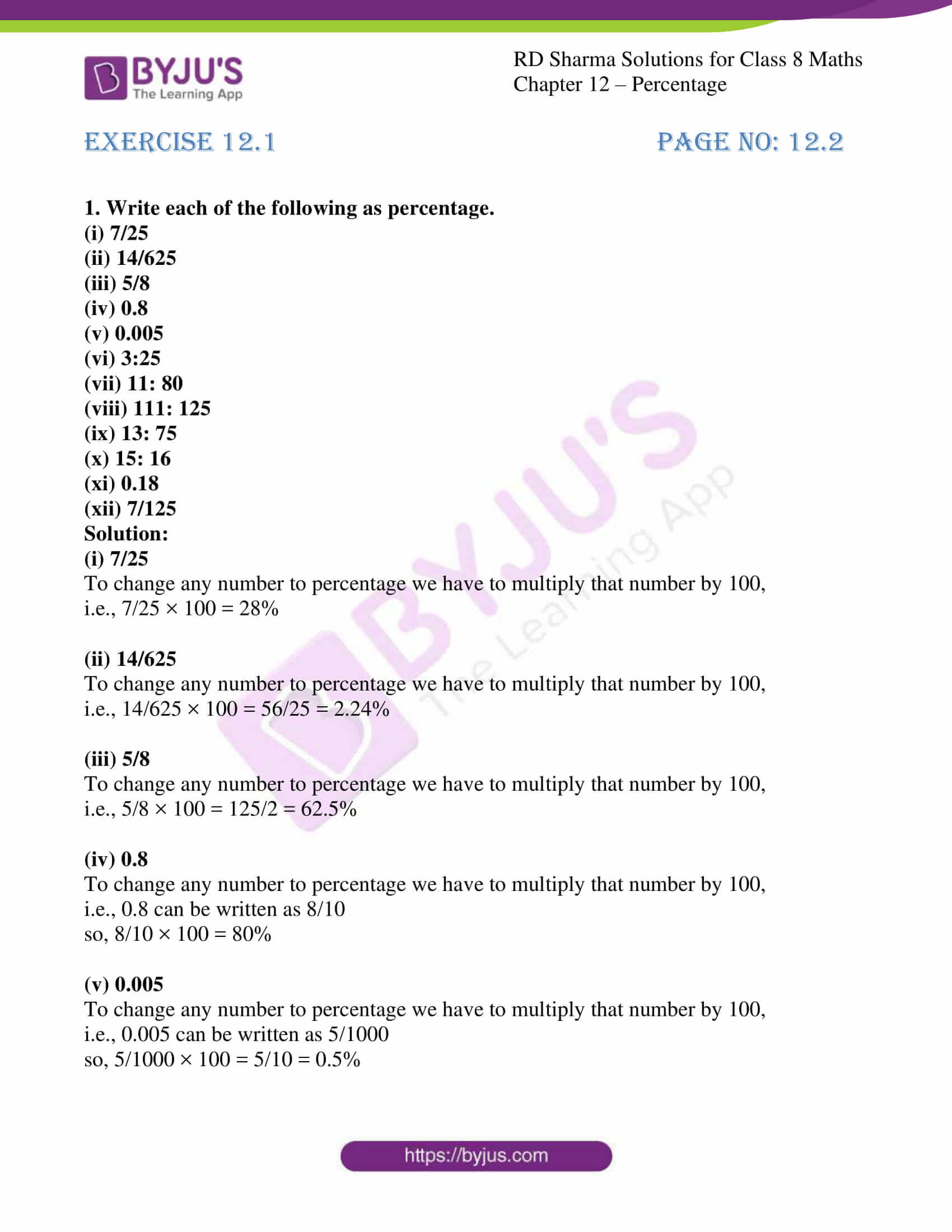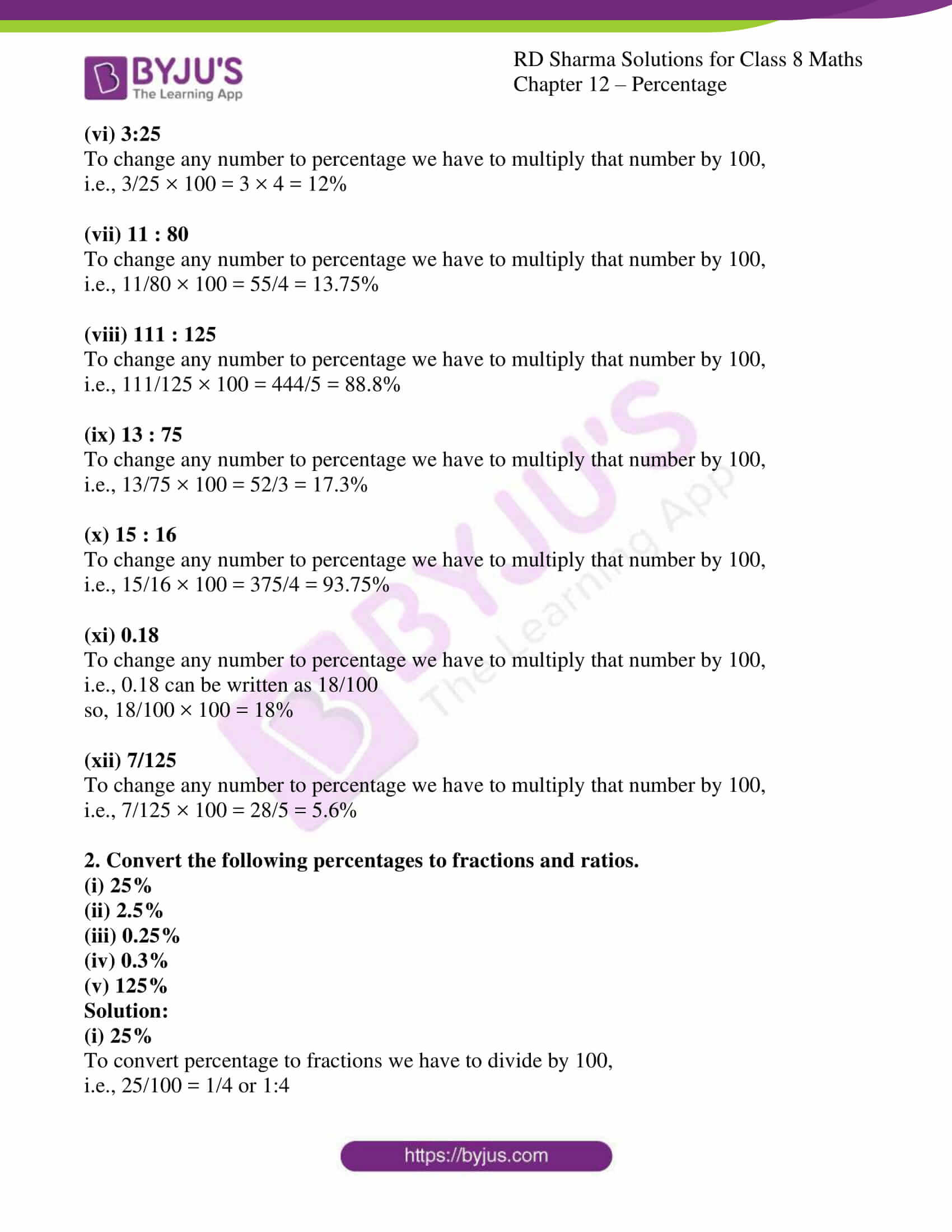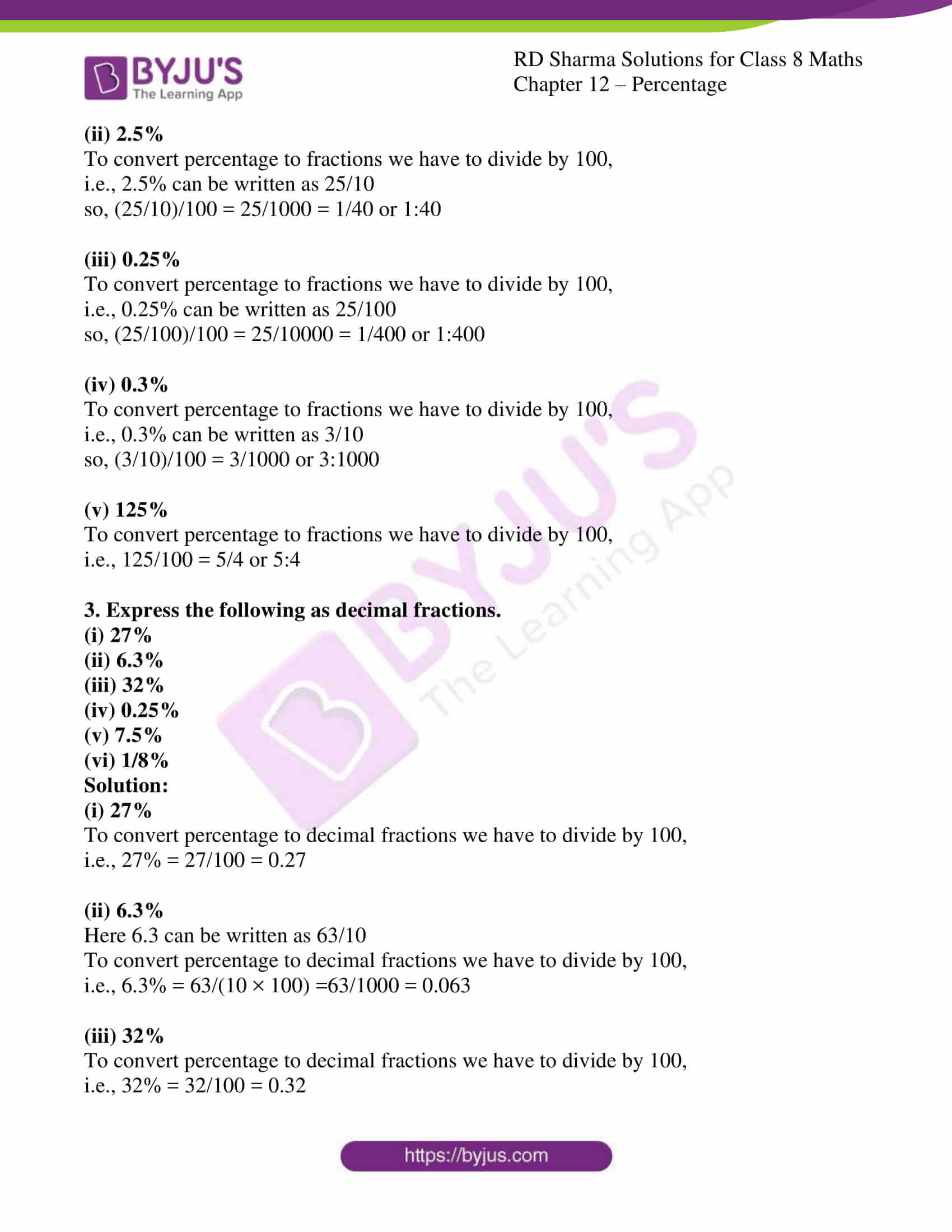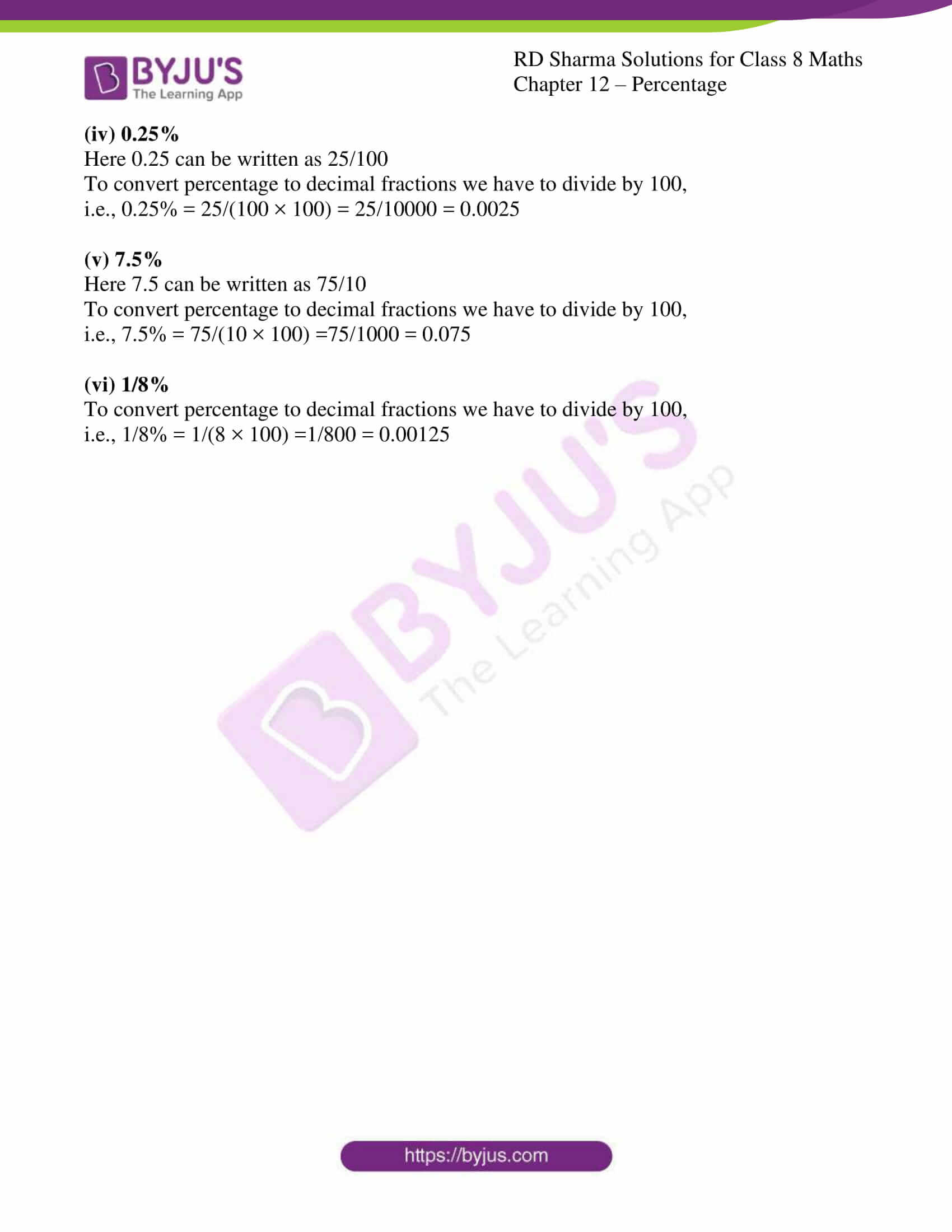# RD Sharma Solutions for Class 8 Maths Chapter 12 Percentage Exercise 12.1

In Exercise 12.1 of Chapter 12, we shall deal with problems based on expressing percentage as a fraction, a ratio, decimal form and vice-versa. Students who are preparing for their exams and who wish to present their answers without any mistakes can refer to RD Sharma Solutions which is formulated by our experts. On regular practice, students can speed up the method of solving problems by using short-cut tips to secure high marks in their examination. Students can download the pdf from the links provided below.

## Download the pdf of RD Sharma Solutions for Class 8 Maths Exercise 12.1 Chapter 12 Percentage### Access Answers to RD Sharma Solutions for Class 8 Maths Exercise 12.1 Chapter 12 Percentage

1. Write each of the following as percentage.
(i) 7/25
(ii) 14/625
(iii) 5/8
(iv) 0.8
(v) 0.005
(vi) 3:25
(vii) 11: 80
(viii) 111: 125
(ix) 13: 75
(x) 15: 16
(xi) 0.18
(xii) 7/125

Solution:

(i) 7/25

To change any number to percentage we have to multiply that number by 100,

i.e., 7/25 × 100 = 28%

(ii) 14/625

To change any number to percentage we have to multiply that number by 100,

i.e., 14/625 × 100 = 56/25 = 2.24%

(iii) 5/8

To change any number to percentage we have to multiply that number by 100,

i.e., 5/8 × 100 = 125/2 = 62.5%

(iv) 0.8

To change any number to percentage we have to multiply that number by 100,

i.e., 0.8 can be written as 8/10

so, 8/10 × 100 = 80%

(v) 0.005

To change any number to percentage we have to multiply that number by 100,

i.e., 0.005 can be written as 5/1000

so, 5/1000 × 100 = 5/10 = 0.5%

(vi) 3:25

To change any number to percentage we have to multiply that number by 100,

i.e., 3/25 × 100 = 3 × 4 = 12%

(vii) 11 : 80

To change any number to percentage we have to multiply that number by 100,

i.e., 11/80 × 100 = 55/4 = 13.75%

(viii) 111 : 125

To change any number to percentage we have to multiply that number by 100,

i.e., 111/125 × 100 = 444/5 = 88.8%

(ix) 13 : 75

To change any number to percentage we have to multiply that number by 100,

i.e., 13/75 × 100 = 52/3 = 17.3%

(x) 15 : 16

To change any number to percentage we have to multiply that number by 100,

i.e., 15/16 × 100 = 375/4 = 93.75%

(xi) 0.18

To change any number to percentage we have to multiply that number by 100,

i.e., 0.18 can be written as 18/100

so, 18/100 × 100 = 18%

(xii) 7/125

To change any number to percentage we have to multiply that number by 100,

i.e., 7/125 × 100 = 28/5 = 5.6%

2. Convert the following percentages to fractions and ratios.
(i) 25%
(ii) 2.5%
(iii) 0.25%
(iv) 0.3%
(v) 125%

Solution:

(i) 25%

To convert percentage to fractions we have to divide by 100,

i.e., 25/100 = 1/4 or 1:4

(ii) 2.5%

To convert percentage to fractions we have to divide by 100,

i.e., 2.5% can be written as 25/10

so, (25/10)/100 = 25/1000 = 1/40 or 1:40

(iii) 0.25%

To convert percentage to fractions we have to divide by 100,

i.e., 0.25% can be written as 25/100

so, (25/100)/100 = 25/10000 = 1/400 or 1:400

(iv) 0.3%

To convert percentage to fractions we have to divide by 100,

i.e., 0.3% can be written as 3/10

so, (3/10)/100 = 3/1000 or 3:1000

(v) 125%

To convert percentage to fractions we have to divide by 100,

i.e., 125/100 = 5/4 or 5:4

3. Express the following as decimal fractions.
(i) 27%
(ii) 6.3%
(iii) 32%

(iv) 0.25%
(v) 7.5%
(vi) 1/8%

Solution:

(i) 27%

To convert percentage to decimal fractions we have to divide by 100,

i.e., 27% = 27/100 = 0.27

(ii) 6.3%

Here 6.3 can be written as 63/10

To convert percentage to decimal fractions we have to divide by 100,

i.e., 6.3% = 63/(10 × 100) =63/1000 = 0.063

(iii) 32%

To convert percentage to decimal fractions we have to divide by 100,

i.e., 32% = 32/100 = 0.32

(iv) 0.25%

Here 0.25 can be written as 25/100

To convert percentage to decimal fractions we have to divide by 100,

i.e., 0.25% = 25/(100 × 100) = 25/10000 = 0.0025

(v) 7.5%

Here 7.5 can be written as 75/10

To convert percentage to decimal fractions we have to divide by 100,

i.e., 7.5% = 75/(10 × 100) =75/1000 = 0.075

(vi) 1/8%

To convert percentage to decimal fractions we have to divide by 100,

i.e., 1/8% = 1/(8 × 100) =1/800 = 0.00125